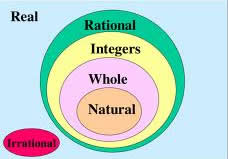# Difference between Real Number and Rational Number

##### Key Difference: A real number is a number that can take any value on the number line. They can be any of the rational and irrational numbers. Rational number is a number that can be expressed in the form of a fraction but with a non-zero denominator. Rational numbers are a subset of the real numbers.Real numbers consist of all the rational as well as irrational numbers. The system of real numbers can be further divided into many subsets like natural numbers, whole numbers and integers.

Natural Numbers (1, 2, 3,….)

Whole Numbers (0, 1, 2, 3, 4, 5,…)

Integers (….., -3, -2, -1, 0, 1, 2, 3,…..)

A real number refers to any number that can be found on the number line. The Number line can be expressed as an actual geometric line where a point is chosen to be the origin. Points that fall in the right side of origin are considered as positive numbers, whereas numbers lying in the left side of origin are considered to be negative. Therefore, they consist of whole (0, 1 ,3 ,9, 26), rational (6/9, 78.98) and irrational numbers (square root of 3, pi). Infinity does not fall in the category of real number. Square root of -1 is also not a real number, and therefore it is referred to as an imaginary number.

A rational number is a number that is determined by a ratio defined as (p/q), where p stands for some integer and q stands for some non-zero natural number. These numbers form a subset of real numbers. On the other hand, real numbers that cannot be expressed as the ratio of two integers are known as irrational numbers. Rational numbers are resulted from theoretical calculation or a definition.

Comparison between Real Number and Rational Number:

 Real Number Rational Number Definition A real number is a number that can take any value on the number line. It can be any of the rational and irrational numbers. Rational number is a number that can be expressed in the form of a fraction but with a non-zero denominator. Number line Can be plotted on the number line. Can be plotted on the number line Includes This includes (but is not limited to) positives and negatives, integers and rational numbers, square roots, cube roots , π (pi), etc. 8, as 8 can be expressed in the form of (8/1) 3/4 , as it is in a fraction form 0/3, as it in a fraction form Square root of 16, as it would be 4 and that can be expressed as (4/1) .7777777, all repeating decimals are rational. .12, as it can be expressed as 12/10 Important Points to remember The union of the sets of rational numbers and irrational numbers. Real numbers include zero. The set of Rational numbers includes all decimals that possess either a finite number of decimal places or that repeat in the same pattern of digits. For example, 0.1111111… = 1/9 and .245245245…. = 245/999. The set of Natural numbers is a subset of the set of Whole numbers, which is contained in the set of Integers, which is inside of the set of Rational numbers.

Image Courtesy: docstoc.com